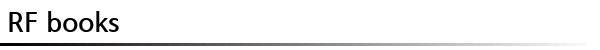Á¶Č¸Ľö : 2009 µµĽ­¸í Introductory Electromagnetics ŔúŔÚ/żŞŔÚ Zoya Popovic, Branko D. Popovic ĂâĆÇ»ç Prentice Hall şĐľß RF/Microwave °ł·Đ ¸ńÂ÷/Ľł¸í Summary Introductory Electromagnetics, Electromagnetic Fields Modern Introductory Electromagnetics is one of the few textbooks suitable for a one-semester course in electromagnetics. It relates basic physical principles to engineering practice with a number of application examples. It is mathematically simple, but exact. -------------------------------- Features Contains application chapters—Which integrate knowledge students gain in previous chapters. Includes a “Questions?feature—Which guides the student as they read the text to provide a qualitative understanding of the material. Derives mathematical tools from physical concepts when needed—Appendices help students who need mathematical review Presents transmission lines in the middle of the text once students have understood distributed circuit elements—The organization draws upon what students have learned in their circuits courses Offers a “Practice Problems and Labs?supplement?Which follows the order of the text) that students can use as a workbook and study guide. --------------------------------------- Table of Contents 1. Electromagnetics Around Us: Some Basic Concepts. 2. Circuit Theory and Electromagnetics. I. TIME-INVARIANT ELECTRIC FIELD. 3. Coulombs Law in Vector Form and Electric Field Strength. 4. The Electric Scalar Potential. 5. Gauss Law. 6. Conductors in the Electrostatic Field. 7. Dielectrics in the Electrostatic Field. 8. Capacitance and Related Concepts. 9. Energy, Forces, and Pressure in the Electrostatic Field. 10. Time-Invariant Electric Current in Solid and Liquid Conductors. 11. Some Applications of Electrostatics. II. TIME-INVARIANT MAGNETIC FIELDS. 12. Magnetic Field in a Vacuum. 13. Magnetic Field in Materials. III. SLOWLY TIME-VARYING ELECTROMAGNETIC FIELDS. 14. Electromagnetic Induction and Faradays Law. 15. Inductance. 16. Energy and Forces in the Magnetic Field. 17. Some Examples and Applications of Time-Invariant and Slowly Time-Varying Magnetic Fields. IV. TRANSMISSION LINES. 18. Transmission lines. V. MAXWELLS EQUATIONS AND THEIR APPLICATIONS. 19. Maxwells Equations. 20. The Skin Effect. 21. Uniform Plane Waves. 22. Reflection and Refraction of Plane Waves. 23. Waveguides and Resonators. 24. Fundamentals of Electromagnetic Wave Radiation and Antennas. 25. Some Practical Aspects of Electromagnetic Waves. Appendix 1: A Brief Survey of Vectors and Vector Calculus. Appendix 2: Summary of Vector Identities. Appendix 3: Values of Some Important Physical Constants. Appendix 4: Electrical Properties of Some Materials at Room Temperature and Low Frequencies. Appendix 5: Magnetic Properties of Some Materials. Appendix 6: Standard (IEC) Multipliers of Fundamental Units. Appendix 7: The Greek Alphabet. Appendix 8: Theory of Lossless Metallic Waveguides. Bibliography. Index. µî·ĎŔÚ admin   ĽöÁ¤ÇĎ±â
 ˇĺ ĂßĂµ/şńĆň(0)
 ¸ń·ĎŔ¸·Î ŔĚ ĂĄŔ» ş¸˝Ĺ şĐŔĚ¶ó¸é ¸ńÂ÷/ ł»żëĂß°ˇĽł¸íŔĚłŞ şńĆň,ĂßĂµ/şńĂßĂµżˇ ´ëÇŃ ŔÇ»ç¸¦ ÇĄ˝ĂÇŘÁÖĽĽżä! ÇŘ´çĽ­Ŕű°ú °ü·ĂµČ ł»żëŔĚ¸é ľî¶˛ ł»żëŔĚµç »ó°üľř˝Ŕ´Ď´Ů. ľĆŔĚµđ :    şńąĐąřČŁ :